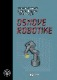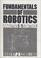### Robotics Practicum

#### Course Description

Robots and robot systems, review. Industrial robots and applications. Structure and control of laboratory SCARA robot. Basic challenges in robot control (direct and inverse kinematics). Introduction to virtual modeling of robot system elements. Control by using Matlab and virtual models. Introduction to robot vision. Intelligent object manipulation.

#### General Competencies

Knowledge about principles and implementations of elements of industrial and mobile robot systems. Understanding of robot control and programming. Understanding of robot vision as a part of applied artificial intelligence. Work with real robot systems and applications.

#### Learning Outcomes

1. differentiate the principles and versions of elements of industrial and mobile robots
2. explain basic types of robot control
3. explain basic principles of robot programming
4. explain basic image processing algorithms for robot vision
5. explain algorithms for object recognition, localization and manipulation
6. create a virtual robot model by using VRML
7. synthesize with VRML robot model by using Matlab
8. explain algorithms for solving direct and inverse kinematics

#### Forms of Teaching

Lectures

Organized in three thematic cycles (5+4+4 hours)

Exercises

Can be organized if students ask for it

Laboratory Work

Two project tasks: • For a given robot task one should design a robot using the LEGO Mindstorm NXT system, build a virtual model and execute the given task in virtual environment using Matlab • For a given robot task one should build a robot using the LEGO Mindstorm NXT system, and write a program and prepare demonstration of robot operation. The robot task assumes the use of robot vision.

Experiments

In-class demonstration of examples of VRML programming.

Consultations

One hour weekly upom request

Seminars

Creation of virtual robot model and control of robot model from Matlab

Acquisition of Skills

Programming of real educational and industrial robots Mastering of basic VRML programming

Other

A multimedia textbook in Croatian is available for students

By decision of the Faculty Council, in the academic year 2019/2020. the midterm exams are cancelled and the points assigned to that component are transferred to the final exam, unless the teachers have reassigned the points and the grading components differently. See the news for each course for information on knowledge rating.

Continuous Assessment Exam
Seminar/Project 0 % 60 % 0 % 60 %
Mid Term Exam: Written 0 % 20 % 0 %
Final Exam: Written 0 % 20 %
Exam: Written 0 % 40 %
Exam: Oral 30 %
##### Comment:

The oral exam share is ±30%.

#### Week by Week Schedule

1. Robots and robot systems - overview.
2. Industrial robots and applications.
3. Structure analysis of a robotic system with a SCARA robot.
4. Kinematics fundamentals - homogeneous coordinates. Robot tool position and orientation.
5. Denavit-Hartenberg method of determining robot kinematic parameters.
6. Direct kinematics. Examples.
7. Inverse kinematics. Examples.
8. Midterm exam
9. Introduction to VRML. Virtual modeling of robotic systems using VRML.
10. Control of virtual robotic systems by using Matlab.
11. Computer tools for modeling, simulation and control of robot systems
12. Introduction to robot vision. Basic algorithms for image processing (noise filtering, edge detection, Hough transformation).
13. Object recognition algorithms (chain code, pattern matching, calculation of moments). Determination of position and orientation of object in the robot workspace.
14. Introduction to intelligent object manipulation using robot vision.
15. Final exam

#### Study Programmes

Control Engineering and Automation (module)
Elective Courses (6. semester)

#### LiteratureZ. Kovačić, S. Bogdan, V. Krajči (2002.), Fundamentals of robotics (in Croatian), Graphis, Zagreb
T. Šurina, M. Crnekovic (1990.), Industrial robots (in Croatian), Školska knjiga, ZagrebR.J. Schilling (1990.), Fundamentals of robotics, Prentice Hall

#### General

ID 34346
Summer semester
4 ECTS
L2 English Level
L1 e-Learning